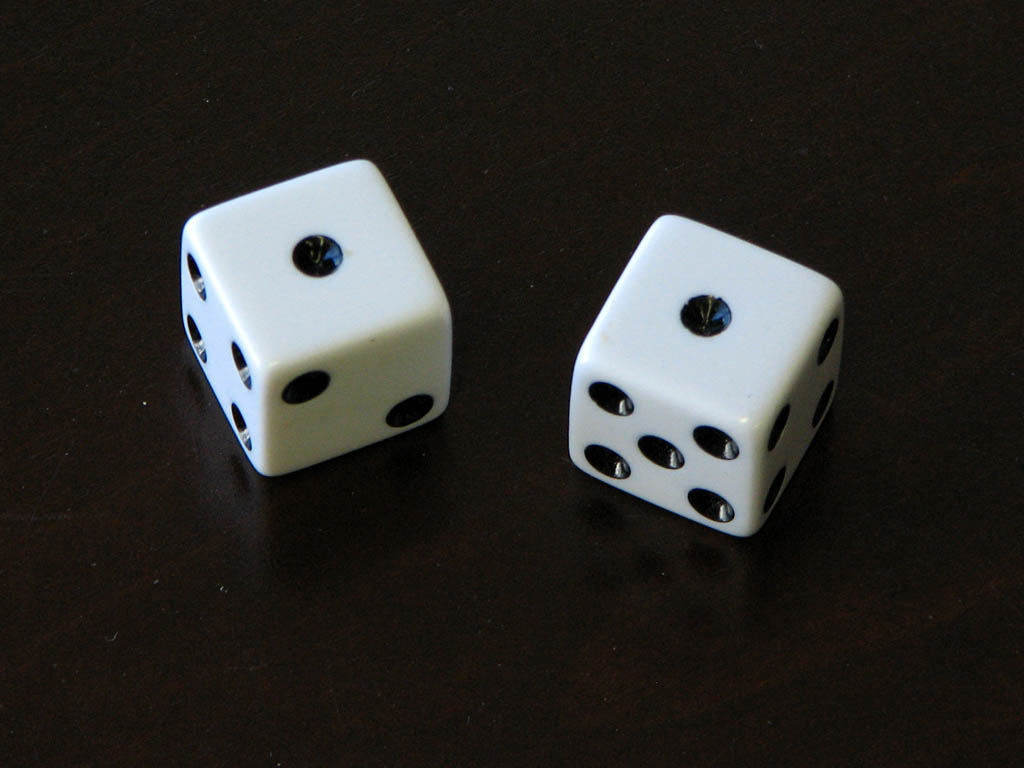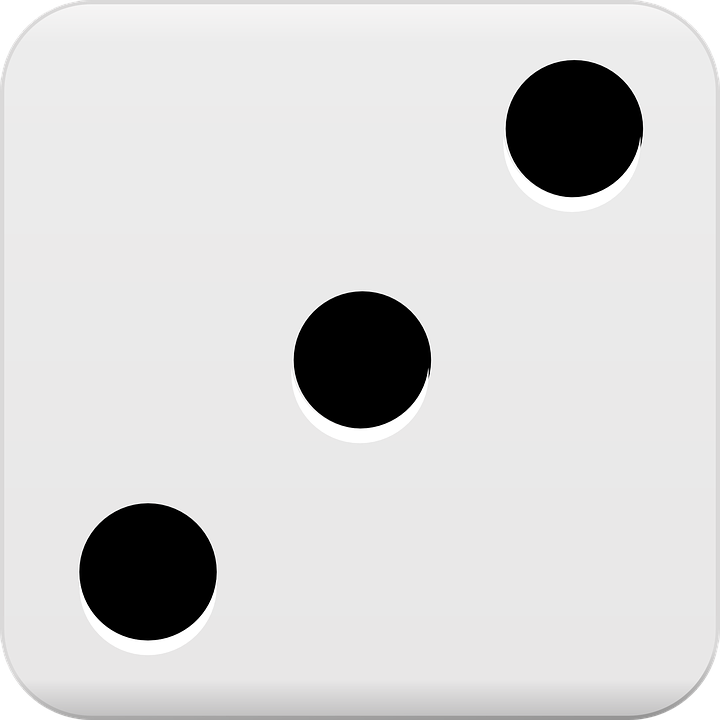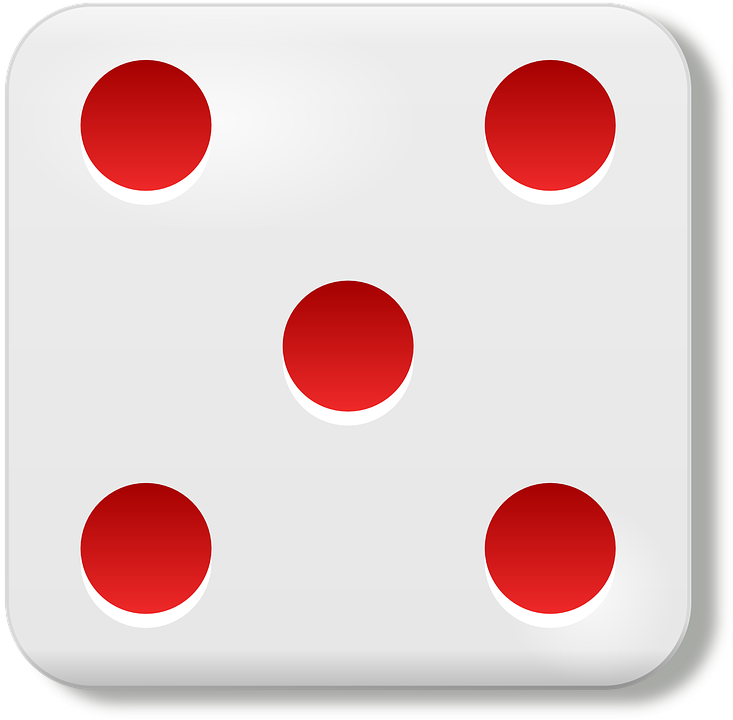## Tuesday, 12 September 2017

### Numbers!

In maths we have been working on a few different things.  In this workshop we were working on estimating and checking by grabbing a handful of matchsticks and guessing how many we thought we had.  Then we counted to check.  We were really trying to get a feel for a number.  Does this feel like a big number or a little number?  Then once we checked and we did it again we could compare and ask ourselves, "does this pile feel bigger or smaller than the last one?" The whole idea was hard because so many of us just wanted to get the "right" answer, but really we were trying to practise feeling the numbers.  This is where growth mindset in maths is so important--it isn't all about knowing the answer or even getting the answer, it's about thinking, questioning and justifying our ideas!

In this workshop we were working on making teen numbers.  We have some confusion around teen numbers (12,13,14,15, 16, 17, etc.) and ty numbers (20, 30, 40, 50, 60, 70, 80, 90) so we are really trying to get to the essence of a teen number and see how teens only have 1 ten and some more, while ty numbers have more bundles of tens.  We are starting to learn that the first number of a 2 digit number shows us how many tens are in the number.

Basic Fact Busses

Our basic fact busses have different information that you can help me with at home.  We are working on trying to instantly recognise numbers and patterns, but one of the main ways we are doing that is through number talks.  We look at the patterns and talk about them.  Children share how they know what the number is without counting all the dots.  For instance, when looking at something like this number pattern one child might explain they knew it was 6, because it's 1 more than 5, or the next number after 5.  Someone else might see it as 3 and 3 and some might say because I know there are 4 missing to make 10, so that gives me 6.  This explanation of how they know and see the numbers helps develop their number sense.  Help your children see these different patterns and help them see there are so many different ways to see 6 and make 6.Here's a look at what different people are working on in their Busses in the next few weeks:
*Please help me practise counting forward and backward (let’s make sure I am saying teen and not ty numbers, e.g. 13 not 30).
 1 + 1 =2 + 2 =3 + 3 =4 + 4 =5 + 5 =6 + 6 =7 + 7 =8 + 8 =9 + 9 =10 + 10 =